# 4. Estimating

Today we are going to be doing something called estimating. We do estimating when we are counting but instead of counting the exact amount we take a clever/sensible guess at how many there are.

We use estimating lots in real life…

• When mummy or daddy are at the supermarket they might estimate how much the shopping is going to cost so they don’t end up spending too much
• When going on a long walk you might estimate the distance you are going to walk so it’s not too short or longLet’s start by having a look at this group of children and adults. How many do you think there are? Can you estimate? It’s really important you DON’T count them as we really want to practise becoming super estimators.

I can see 2 adults straight away and maybe about 5 children. I think my estimate needs to be close to 7.

Do you agree? Why?

How can we check exactly how many there are? Now you can count them.

There are 8. I was very close!

It doesn’t matter that my estimate/clever guess doesn’t match the exact number because we today we are only learning to estimate.

Let’s look at this jar of sweets. I can see straight away there aren’t many. It doesn’t look like a big number.

Can you estimate how many there are?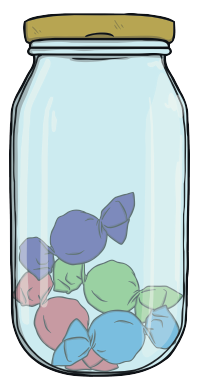Estimate: ____________   Actual amount: ____________

Once you have made an estimate, have a go at counting them.

Now I know what 4 looks like it can help me estimate how many sweets are in the next jar. Are there more or less than 4? Definitely more! Have a go at estimating how many there are. Then count them. Remember, you DON’T have to be the same as the exact amount.

An estimate is a sensible guess!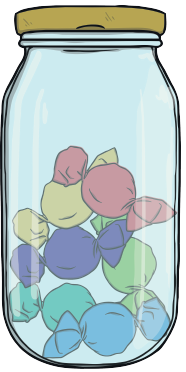Estimate: ____________   Actual amount: ____________

Estimate how many animals are in the field? Have a go at counting them afterwards. Was your estimate sensible?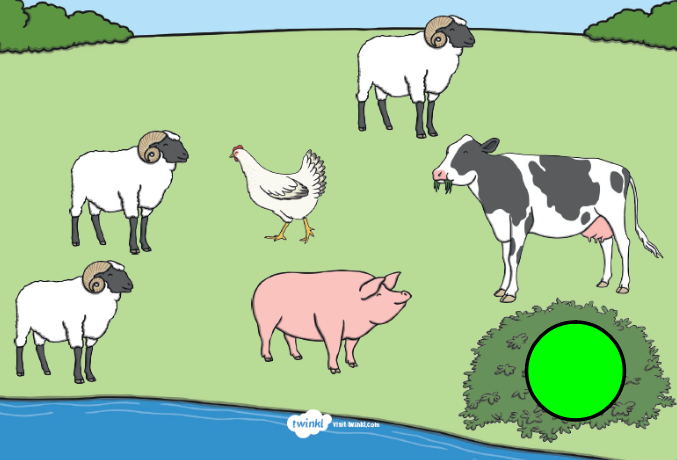Estimate: ____________   Actual amount: ____________

Now that you know what 6 looks like, estimate how many animals there are now.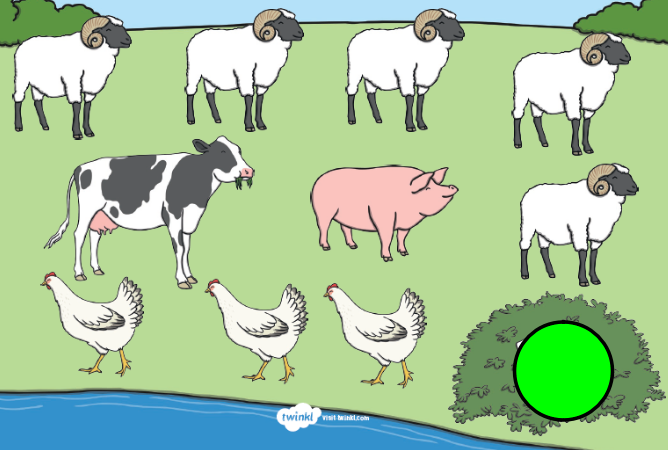Estimate: ____________   Actual amount: ____________

Estimate how many fish are in the tank. I can see 3 yellow fish already so I know the number has to be bigger than 3.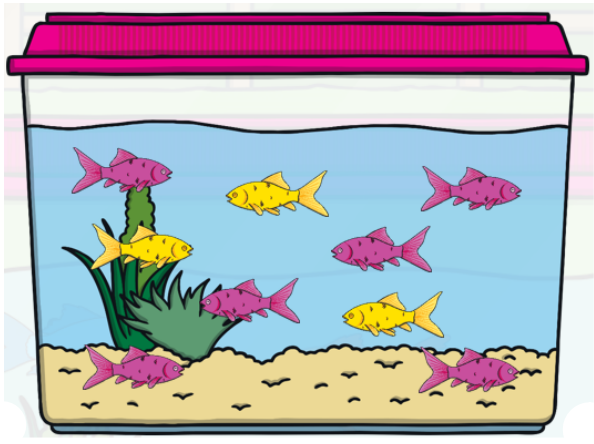Estimate: ____________   Actual amount: ____________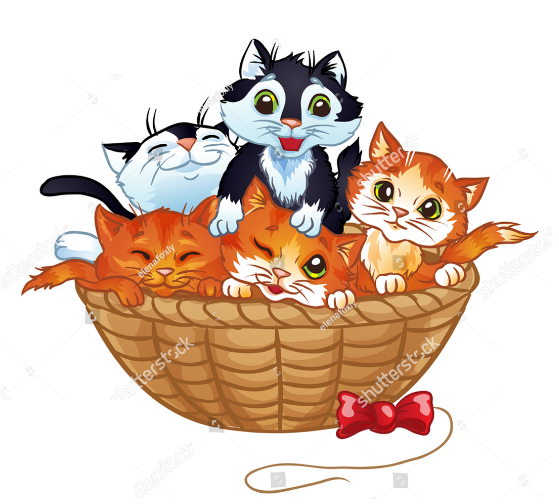Estimate the amount of cats.

 Estimate: ____________   Actual amount: ____________

Remember estimate do not need to be exact.